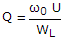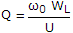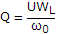# Electronics and Communication Engineering - Microwave Communication

### Exercise :: Microwave Communication - Section 5

1.

In 8 cavity magnetron the p mode corresponds to a total phase shift equal to

 A. ± 8 p radians around the periphery B. ± 4 p radians around the periphery C. ± 2 p radians around the periphery D. ± 16 p radians around the periphery

Explanation:

No answer description available for this question. Let us discuss.

2.

Satellite communication systems use the frequency band

 A. 10 to 20 MHz B. 50 to 70 MHz C. 3 to 6 GHz D. 100 to 120 GHz

Explanation:

No answer description available for this question. Let us discuss.

3.

The characteristic impedance of a cable is about

 A. 300 Ω B. 50 Ω C. 5 Ω D. 2 Ω

Explanation:

No answer description available for this question. Let us discuss.

4.

The Q factor of a waveguide resonator is given by (ω0 is resonant frequency, U is energy storage and WL is the power loss)

 A.B.C. Q = ω0UWL D.Explanation:

No answer description available for this question. Let us discuss.

5.

A TR (transmitter receiver) tube has

 A. low loss for low power signals and high B. high loss for low power signals and low loss for high level signal C. low loss for both low level and high level signals D. high loss for both low level and high level signals